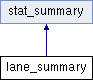lane_summary Class Reference

`#include <lane_summary.h>`

Inheritance diagram for lane_summary:## Public Types

typedef metric_stat metric_stat_t

typedef surface_summary_vector_t::reference reference

typedef surface_summary_vector_t::const_reference const_reference

typedef surface_summary_vector_t::iterator iterator

typedef surface_summary_vector_t::const_iterator const_iterator

typedef surface_summary_vector_t::size_type size_typePublic Types inherited from stat_summary
typedef metric_stat metric_stat_t

## Public Member Functions

lane_summary (const size_t lane=0, const size_t channel_count=0)

surface_summaryoperator[] (const size_t n) INTEROP_THROW_SPEC((model

surface_summaryat (const size_t n) INTEROP_THROW_SPEC((model

iterator begin ()

iterator end ()

const_iterator begin () const

const_iterator end () const

void resize (const size_type n)

void lane (const size_t val)

void tile_count (const size_t val)

cycle_state_summarycycle_state ()

const surface_summaryoperator[] (const size_t n) const INTEROP_THROW_SPEC((model

const_reference at (const size_type n) const INTEROP_THROW_SPEC((model

size_t lane () const

size_t tile_count () const

const cycle_state_summarycycle_state () const

size_t size () constPublic Member Functions inherited from stat_summary
stat_summary (const size_t)

float percent_gt_q30 () const

float yield_g () const

float projected_yield_g () const

const metric_stat_tdensity () const

const metric_stat_tdensity_pf () const

const metric_stat_tcluster_count () const

const metric_stat_tcluster_count_pf () const

const metric_stat_tpercent_pf () const

const metric_stat_tphasing () const

const metric_stat_tprephasing () const

const metric_stat_tpercent_aligned () const

const metric_stat_terror_rate () const

const metric_stat_terror_rate_35 () const

const metric_stat_terror_rate_50 () const

const metric_stat_terror_rate_75 () const

const metric_stat_terror_rate_100 () const

const metric_stat_tfirst_cycle_intensity () const

const metric_stat_tphasing_slope () const

const metric_stat_tphasing_offset () const

const metric_stat_tprephasing_slope () const

const metric_stat_tprephasing_offset () const

const metric_stat_tpercent_occupied () const

void percent_gt_q30 (const float val)

void yield_g (const float val)

void projected_yield_g (const float val)

void density (const metric_stat_t &stat)

void density_pf (const metric_stat_t &stat)

void cluster_count (const metric_stat_t &stat)

void cluster_count_pf (const metric_stat_t &stat)

void percent_pf (const metric_stat_t &stat)

void phasing (const metric_stat_t &stat)

void prephasing (const metric_stat_t &stat)

void percent_aligned (const metric_stat_t &stat)

void error_rate (const metric_stat_t &stat)

void error_rate_35 (const metric_stat_t &stat)

void error_rate_50 (const metric_stat_t &stat)

void error_rate_75 (const metric_stat_t &stat)

void error_rate_100 (const metric_stat_t &stat)

void first_cycle_intensity (const metric_stat_t &stat)

void phasing_slope (const metric_stat_t &stat)

void phasing_offset (const metric_stat_t &stat)

void prephasing_slope (const metric_stat_t &stat)

void prephasing_offset (const metric_stat_t &stat)

void percent_occupied (const metric_stat_t &val)

void resize_stat (const size_t)

## Friends

template<class MetricType , int Version>
struct io::generic_layout

## Detailed Description

Summary statistics compiled by lane

Note
The reported standard deviation is always between tiles, not cycles. For metrics like error metrics, the mean over all cycles is used for each tile, before calculating the standard deviation.

## Member Typedef Documentation

 typedef surface_summary_vector_t::const_iterator const_iterator

Constant random access iterator to vector of lane_summary

 typedef surface_summary_vector_t::const_reference const_reference

Constant reference to lane_summary

 typedef surface_summary_vector_t::iterator iterator

Random access iterator to vector of lane_summary

 typedef metric_stat metric_stat_t

Collection of metric statistics type

 typedef surface_summary_vector_t::reference reference

Reference to lane_summary

 typedef surface_summary_vector_t::size_type size_type

Unsigned integral type (usually size_t)

## Constructor & Destructor Documentation

 lane_summary ( const size_t lane = `0`, const size_t channel_count = `0` )
inline

Constructor

Parameters
 lane lane number channel_count channel count

## Member Function Documentation

 surface_summary& at ( const size_t n )
inline

Get reference to lane_summary at given index

Deprecated:
Will be removed in next feature version (use operator[] instead for C++ Code)
Parameters
 n index
Returns
reference to lane_summary
 iterator begin ( )
inline

Get random access iterator to start of summaries by lane

Returns
random access iterator
 const_iterator begin ( ) const
inline

Get random access iterator to start of summaries by lane

Returns
random access iterator
 cycle_state_summary& cycle_state ( )
inline

Get statistics summarizing the cycle of each RTA state of tiles in the lane

Returns
statistics summarizing the cycle of each RTA state of tiles in the lane
 iterator end ( )
inline

Get random access iterator to end of summaries by lane

Returns
random access iterator
 const_iterator end ( ) const
inline

Get random access iterator to end of summaries by lane

Returns
random access iterator
 void lane ( const size_t val )
inline

Set lane number

Parameters
 val lane number
 surface_summary& operator[] ( const size_t n )
inline

Get reference to lane_summary at given index

Parameters
 n index
Returns
reference to lane_summary
 void resize ( const size_type n )
inline

Resize the summary by lane vector

Parameters
 n new size of summary by lane vector
 void tile_count ( const size_t val )
inline

Set number of tiles in the lane

Parameters
 val number of tiles in the lane

## Friends And Related Function Documentation

 friend struct io::generic_layout
friend

The documentation for this class was generated from the following file: